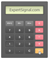Defining Oscillators

Oscillators are technical analysis tools that bound within a predefined range. Oscillators are placed below the main chart area and not within the chart area as common indicators.Oscillators are tools of technical analysis that bound within a range. This predefined range helps traders to identify if the price of a financial asset is found in overbought/oversold levels.

Oscillators can prove a useful tool for:

• Identifying Overbought/Oversold Market Levels
• Evaluating the Momentum of the Trend
• Spotting Trend Reversal/Continuation

But how easy it is to spot a failure in the momentum of the trend?

“When a long-term trend loses its momentum, short-term volatility tends to rise. It is easy to see why that should be so: the trend-following crowd is disoriented.” -George Soros

This tutorial includes information and tips when trading with the following oscillators:

Commodity Channel Index

(CCI)

Usage: Identifying Overbought/Oversold Markets

Standard Settings: 14 Periods (Recommended 20 Periods)Introduction to CCI

The Commodity Channel Index or CCI is an oscillator that aims to identify overbought/oversold market levels. CCI is able to measure the current position of a financial asset with respect to its moving average. CCI can also signal trades as you can see below, these signals are more reliable in ranging markets.Calculating CCI

CCI = (TP - SMA (20) of TP) / (Constant x Mean Deviation)

Where:

• TP (Typical Price) = (High + Low + Close) / 3
• Constant = .015

%R Larry Williams

(Williams %R)

Usage: Identifying Overbought/Oversold Market Levels

◙ Trading: Detecting Trend Continuation / Momentum Failures

Standard Settings: 14 PeriodsIntroduction to Williams %R

Developed by Larry Williams, Williams %R is a momentum oscillator widely used for its ability to identify overbought and oversold market levels and to detect momentum failures.

-Williams %R oscillates from values 0 to -100

-Williams %R shows the level of the close relative to the highest high for a particular period:

• When Williams %R is near zero (0), it shows the price is trading near the highest high (look back period)
• When Williams %R is near -100, it shows the price is trading near the lowest low (look back period)

-Similarly, the Stochastic Oscillator shows the level of the close relative to the lowest low (► more about Stochastic)

-Williams %R and the Fast Stochastic Oscillator produce the exact same reading, only the scaling is differentRelative Strength Index

(RSI)

Usage: Identifying Overbought/Oversold Markets

Standard Settings: 14 Periods (we recommend 21 periods)Introduction to RSI

Developed by Welles Wilder, the Relative Strength Index or RSI is a momentum oscillator measuring the velocity and magnitude of directional price movements. The RSI is known for its ability to identify overbought and oversold markets but also to generate trading signals.Calculating RSI

■ RSI = 100 – 100 / ( 1 + RS)

Where,

RS = Average Gain / Average Loss

■ First RS calculation:

Average Gain = Summary of Gains for 14 periods / 14

Average Loss = Summary of Losses for 14 periods /14

■ Second RS calculation:

Average Gain = [(previous Average Gain) * 13 + current Gain] / Average Loss = [(previous Average Loss) * 13 + current Loss] /

■ Same procedure for the third and the subsequent RSTrading with RSI

RSI can be used for multiple purposes:

• Identifying Overbought/Oversold Markets
• Trading Signals on Slopes Divergences (price chart slope vs RSI slope)

Moving Average Convergence Divergence

(MACD)

Usage: Trend-Following Momentum Indicator

Standard Settings: 12-period Fast, 26-periods Slow, and 9-period EMAIntroduction to MACD (Moving Average Convergence Divergence)

Developed by Gerald Appel in the 1970s, MACD is a trend-following momentum indicator and one of the most widely used technical analysis indicators worldwide. The oscillator can evaluate the momentum of the trend and generate reliable trading signals.

• The MACD is the difference between a 26-day and 12-day EMA (exponential moving average)
• The Signal Line is a 9-day EMA which works a trigger for buying/selling the market

The MACD can be used for trading any financial market (Foreign Exchange, Equities, Commodities) in multiple timeframes. There are two ways to visualize MACD:

(a) 2 classic lines system (MACD and Signal Line) and focus on where they are interacting

(b) MACD Histogram (and focus on crossovers above/below zero)

The MACD Histogram

Developed by Thomas Aspray, the MACD-Histogram shows the distance between the MACD line and the signal line.Stochastic Oscillator

Usage: Evaluating the Trend and Detecting Overbought/Oversold Markets

Standard Settings: 14 Periods (14,3,3)Introduction to the Stochastic Oscillator

Developed by George Lane, the 'Stochastic' is an oscillator that can measure the momentum and the speed of a price. It can be used as a tool for identifying trend reversals or trend continuation.

The Stochastic bound between 0 and 100 and it can identify overbought and oversold market levels as follows:

□ Overbought market levels are set at reading 80

□ Oversold market levels are set at reading 20Calculating the Stochastic Oscillator

The Stochastic is formed by two lines

■ %K = {(Last Close - Lowest Low in K period)/(Highest High in K period - Lowest Low in K period)} * 100

Where,

K is the number of periods. Standard settings K=14 (14,3,3)Trading with the Stochastic Oscillator

The Stochastic can be used for:

• Detecting Overbought/Oversold Markets
• Identifying Trend Reversal/Continuation